The General Linear Model
The garden variety linear model can be written as
Y = Xb + U
A. Assumptions
1. The design matrix, n observations on each of k variables, is fixed in repeated samples of size n. This implies that X : nxk is not stochastic. Also, n > k.
2. The n x k design matrix is of full column rank. That is, the columns of the design matrix are linearly independent. The implication is that the columns of X form a basis for a k-dimension vector space.
3.a. The n-dimension disturbance vector U consists of n i.i.d. random variables such that
E(U) = 0
E(UU') = s2In
where s2 is an unknown parameter.
Or,
b. The disturbance vector is an n-variate normal r.v.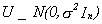The assumption that the design matrix be non stochastic is unnecessarily stringent. We only need assume that the disturbances and the independent variables are independent of one another.
B. Statement of the Model
Let Y be an n x 1 vector of observations on a dependent variable. For example, the crime rate in each of a number of communities at a point in time, or in one community over a number of time periods.
Let X be n observations on each of k independent variables, n>k. For example, distance of community from the urban center, relative wealth of the community, and probability of apprehension.
While Y is an n-dimensional vector, we have only k variables to explain it. This leaves us with two observations. First, we have too many equations, n, and too few unknowns, k. Second, we will need a rule for mapping the n-vector into the k-dimensional space spanned by the columns of X.
We postulate the following linear model
Y = X
b + U
where b is a k x 1 parameter vector and U is an n x 1 disturbance vector. b is not observable.

C. Least Squares Estimation of the Slope Coefficients
1. The Estimator
We wish to choose b to minimize the sum of squared deviations between the observed values of the dependent variable and the fitted values for our given data on X. That is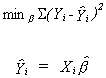Xi denotes the ith realization of the k independent variables.
In vector notation we wish to minimize, by our best guess for the unknown parameter vector, a quadratic form which we will denote by Q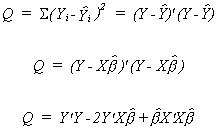We proceed in the usual fashion by deriving the k first order conditions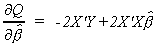Set each of the equations to zero and solve for the unknown parameters.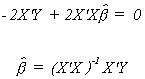Note that in solving the system of equations for the k unknowns it was critical that the columns of X be linearly independent. Were they not independent it would not have been possible to construct the necessary inverse.
2. The Mean of the Estimator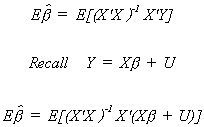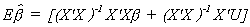We should note several things: Expectation is a linear operator. The error term is assumed to have a mean of zero. X'X cancels with its inverse. Initially we assumed that X is non-stochastic. So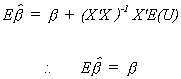The least squares estimator is linear in Y, and by substitution it is linear in the error term. It is also unbiased.

3. The Variance of the Estimator
Our starting point is the definition of the variance of any random variable.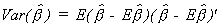Substituting in from the expression for the mean of the parameter vector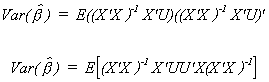Again, since the X are non-stochastic and expectation is a linear operator we can cut right to the heart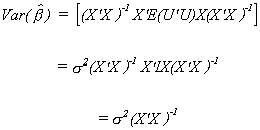4. The Gauss Markov Theorem
We now come to one of the simpler and more important theorems in econometrics. The Gauss-Markov Theorem states that in the class of linear unbiased estimators the OLS estimator is efficient. By efficient we mean that estimator with the smallest variance in its class.
Theorem
If r(X) = k where X: nxk
E(U) = 0 E(UU')=s2I
then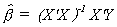is BLUE.
Proof:
We seek an estimator b* that is a linear unbiased estimator with a smaller variance than the OLS estimator.
Let b* = C*Y be an arbitrary linear estimator. Since this is a linear estimator and OLS is a linear estimator we can choose C* to be a combination of the design matrix. Namely, C* = C + (X'X)-1X'.
As before Y = Xb + U.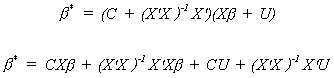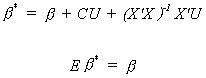For b* to be unbiased we must impose the restriction that CX = 0. Now from the definition of variance and using the fact that our new estimator is unbiased we have Var(b*) = E(b* - b)(b* - b)'. Or, substituting in for b*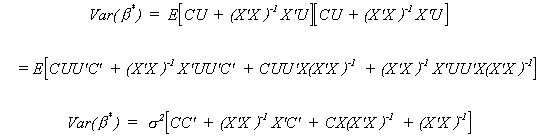But we already know CX = X'C' = 0 so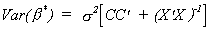Since CC' must be positive semi-definite we know
Var(b*) � Var()
D. Estimation of the Variance of the Error Vector
We will construct our estimator by analogy. Our assumption was that E(UU') = s2I; or, EUi2 = s2 for i=1,2,..,n. It seems plausible then that we use the sum of squared residuals from our least squares estimator in the construction of an estimate of the error variance. Letting e denote the least squares residual, the sum of squared residuals is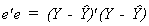Dividing this by n-k gives us our estimator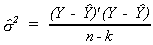There is some logic to this choice of denominator. As we shall see, it gives us an unbiased estimator. Second, there are n observations and we have already used them to compute k estimates, so should be penalized accordingly. Third, because of the method of construction of the estimator of b, we can freely choose only n-k of the least squares residuals. Furthermore, the numerator has a chi-square distribution with n-k degrees of freedom.
Before taking expectations we will substitute in for Y and for the fitted values of Y.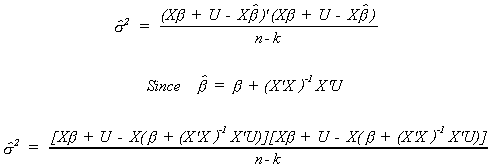With a bit of algebra we can write this as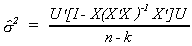This is clearly a scalar. Taking the trace changes nothing, but allows us to change the order of multiplication.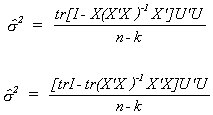We know that EUU' = s2I. The trace of the first identity matrix is n, the second trace is k. Therefore, we conclude that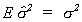E. Maximum Likelihood Estimation
Recall that in our development of the OLS estimator we made the following assumptions:
1. E(U) = 0
2. E(UU') = s2I
3. X is fixed in repeated samples of size n
4. r(X) = k < n; X is of full column rank
and from these assumptions we can show that OLS is BLUE.
Suppose that we replace the first two assumptions by
U ~ N(0,s2I).
With this assumption we can construct the maximum likelihood estimator.
The intuition behind the maximum likelihood estimator is that knowing the distribution function that generated the data and having a sample in hand, we must pick estimates of the distribution parameters which make it most likely to have observed the particular sample. Consider the following parable: The Queen has a son in need of a spouse. She issues a proclamation stating that whosoever is able to guess the number of balls in an urn by her throne may have the son in marriage. The balls in the urn are numbered consecutively from one. Suitors are permitted to reach into the urn and withdraw a ball prior to making their guess. If the guess is incorrect then the suitor is fed to the lions. Ghislain, from Proven�e, wants to take her chances. What should be her rule for construction of a maximum likelihood estimate? Suppose she reaches into the urn and withdraws a ball with the number 59 on it. Would she ever guess 100 balls in the urn? The answer is no. If there are 100 balls in the urn the probability of drawing number 59 is only 1/100. If there are 59 balls in the urn then the probability of drawing number 59 rises to 1/59.
Returning now to regression analysis, the joint density of U, the disturbance vector is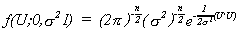But note that U = Y - Xb. Making this substitution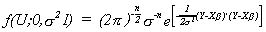Reasoning from the parable recited above, what set of b and s2 will give the greatest likelihood of having observed the particular disturbance vector?
We write the likelihood function as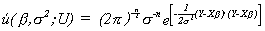We wish to maximize this function by our choice of b and s2. This requires setting the first derivatives of �(.) with respect to b and s2 equal to zero. Since exponents are tough to deal with we will take logs. The log likelihood function is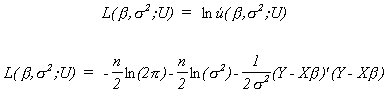Let us expand the last term to get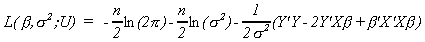Now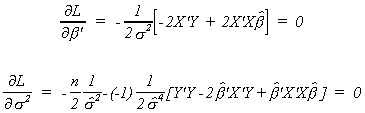Solving the first k equations for b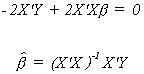The last equation is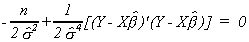Multiply through by 2s4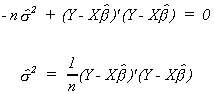If we use our previous convention of e denoting the fitted residuals we can write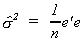While this is similar to our previous result, it is clear that this is not an unbiased estimator of the error variance. It is however, consistent, which we prove elsewhere in the lecture notes. Recall that we had defined consistency as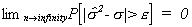That is to say, the probability that our estimator differs from the population parameter by more than an arbitrarily small number can be driven to zero by increasing the sample size.
Since the maximum likelihood estimator is the same as the OLS estimator and U ~ N(0,s2I) we conclude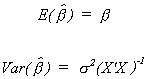Furthermore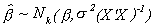We previously proved that OLS is BLUE. As a result of our stronger assumption for deriving the MLE we can make a stronger statement about the behavior of . The following theorem has been provided by C.R. Rao.
THEOREM
If Y = Xb + U and U ~ N(0,s2I), then the maximum likelihood estimatoris the best (minimum variance) estimator in the class of unbiased estimators.

This is a stronger result than Gauss-Markov since we have broadened our attention to include both linear unbiased and nonlinear unbiased estimators.
F.1. Hypothesis Testing
1. General Remarks
Let us assume U ~ N(0,s2I). We had adopted the notation for our model Y = Xb + U and the least squares residuals e = Y - X. The OLS estimator is = (X'X)-1X'Y. Making the obvious substitutions allows us to write
e = Y - X(X'X)-1X'Y
e = (I - X(X'X)-1X')Y
e = (I - X(X'X)-1X')(Xb + U)
e = (I - X(X'X)-1X')Xb + (I - X(X'X)-1X')U
The first term is zero, so e = (I - X(X'X)-1X')U. Recall from the linear algebra chapter that (I - X(X'X)-1X') is an idempotent matrix of order nxn. But what of its rank?
Rank(I - X(X'X)-1X') = Rank(I) - Rank(X(X'X)-1X')
The identity matrix is rank n. The rank of X is k so the rank of the second term is also k. So we are left with the following conclusion
e'e =U'(I - X(X'X)-1X')'(I - X(X'X)-1X')U
e'e = U'(I - X(X'X)-1X')U ~ s2c2n-k
In what follows we will adopt the following naming and notation conventions:
e'e is often called the residual sum of squares, RSS
Y'Y is often called the total sum of squares, TSS
Y'Y - e'e is the estimated or explained sum of squares, ESS
Depending on the objective, the explained sum of squares is often written in several other forms: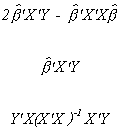These are all found by substitution for the definition of Y and/or beta hat.
We now have sufficient machinery to test some hypotheses.
2. Student's t test
Define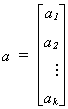In general we may wish to test the hypothesis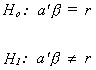where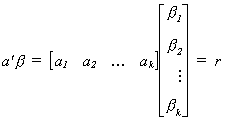Some specific examples include the following
1. Choose a1 = 1 , ai = 0 for i=2,3,...,k, r=0. Then the null is that b1 = 0.
2. Choose ai = 1 , aj = 0 for j � i and r = 0. Then the null is that bi = 0.
3. Choose ai = 1 " i. Then the null is that Sbi = r.
4. Choose ai = 1 , aj = -1 , all other al = 0. Then the null is that bi - bj = 0.
As a test statistic for the general form of the hypothesis test we propose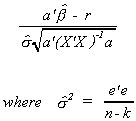The a' and a in the denominator pick off the appropriate elements of the covariance matrix of the estimator to construct the variance of the linear combination of random variables in the null hypothesis.
It can be shown that if U ~ N(0,s2I) then under the null hypothesis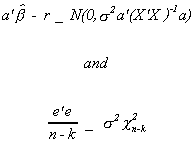Furthermore, these two random variables are independent of one another. Therefore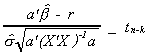3. The F test
It may be the case that we wish to do more than conduct tests of hypothesis on each of the bi or some linear combination of them. We might want to construct a joint test involving M � k of the model coefficients.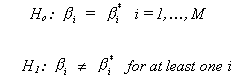Let us adopt the following notation
W: denotes the complete model or the maintained hypothesis
w: denotes a simplified model, in which the parameters of the model satisfy the constraints as specified in the null hypothesis.
For instance, our maintained model might be
Y = b1 + b2X2 + . . . + bkXk + U
and would be named W. Our simplified model might be
Y = b1 + U
and would be named w. The implication of these two models is that quite possibly only the intercept matters. In this example we would retrieve the residual sum of squares from the regression results on the maintained and simplified model. That is,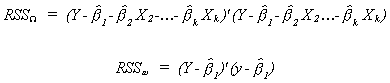Using the notation we have adopted so far, a typical Analysis of Variance (ANOVA) table, from standard regression routines, for the null that all of the slope coefficients are zero is

o TSS-RSSw is the variation in Y explained by the intercept.
o RSSw-RSSW is the variation in Y, beyond that explained by the mean of , explained by the independent variables.
o RSSW is the variation in Y left over after taking account of the intercept and slope parameters.
For the example we are now doing
Ho: b2 = ... = bk = 0
H1: bi � 0 for some i
we would construct the test statistic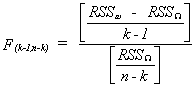Notice that the degrees of freedom in the numerator is the number of variables restricted to zero in going from the complicated model to the simple model. If the observed F is larger than an appropriate critical F then we must conclude that one, or some, or all of the bi i = 2,...,k are not zero.
EXAMPLE
Suppose we had the following model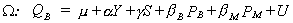and we wish to test the hypothesis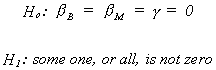The restricted version of the model would be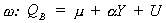To construct the test statistic first estimate W and find the sum of squared errors (RSSW). Then estimate w and find RSSw. Construct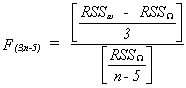F.2. Restricted least Squares
In the previous section we relied on the "restricted model" in doing both the F and t-test. Just how does one construct the restricted model estimates when the restrictions involve more than simple zero restrictions?
We have the model y = X + u with E(u X)= 0, E(u'X) = 0 and Euu'=2I. In addition we have some prior information in the form of J<k exact, independent, linear restrictions R-r=0. Previously we had chosen a best guess for using the principle of least squares. With the addition of the restrictions we must now use the method of Lagrange multipliers.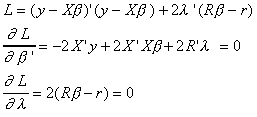The first k first order conditions can be rearranged as follows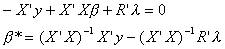From the same first order conditions we can also write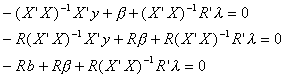Substituting r = R from the last J first order conditions we have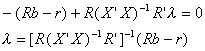So the restricted least squares estimator is given by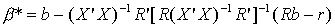The estimator is just a linear combination of the ordinary least squares estimator, b. That is, we need only run the unrestricted regression, then do a few computations to get the restricted estimates.
The mean of this estimator is seen to be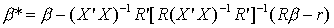So the restricted least squares estimator is unbiased only when the linear restrictions are identically correct.
The variance of the estimator is found as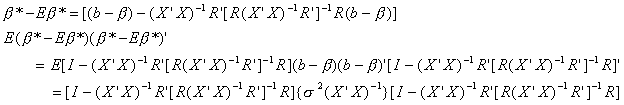Doing the multiplication and exploiting the properties of idempotent matrices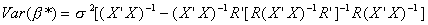Both terms in square brackets are positive definite, since they are both variances. The first term is the OLS covariance matrix, from which we are subtracting another positive definite matrix. The result is positive definite since it is a covariance matrix. Clearly then the restricted least squares estimator has a smaller variance than its unrestricted counterpart. However, this is not the end of the story. Although it has a smaller variance, the restricted estimator may be biased, so they choice of estimator is not so simple. We will revisit this question in the section which reviews hypothesis testing and model selection in multiple regression.

G. Confidence Ellipses and Intervals
1. Confidence Interval for One Parameter
Constructing a confidence interval for one parameter in a regression model is done analogously to the procedure you used for constructing confidence intervals for the mean of a random variable. Assume first that U ~ N(0,s2In). Then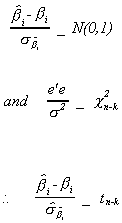Note that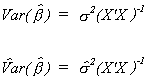so we can write the following probability statement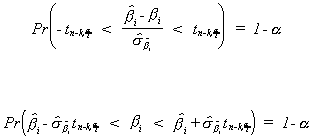2. Confidence Ellipse for a Set of Parameters
Just as the confidence interval is the dual to the test of significance of a single parameter, the confidence ellipse is the dual to an F-test. Suppose there is a set of variables for which we wish to construct a joint confidence interval. Begin by reordering and partitioning the slope coefficients so that the M which interest us are in the top part of the coefficient vector: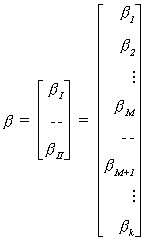Then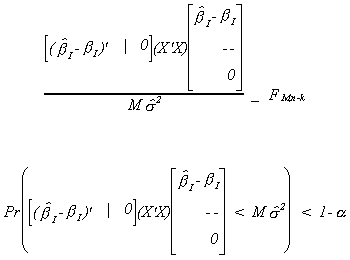For the case where M=2 we get a result that looks like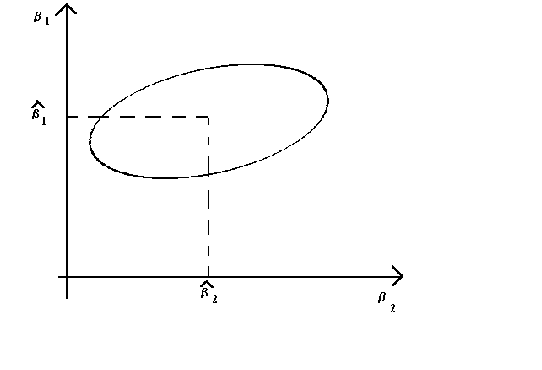1
The elongation and tilt of the ellipse are determined by the quadratic form (X'X). By finding the characteristic roots and vectors of X'X one can first tilt the ellipse to one of the axes then squash it into a circle. See your homework assignment from the linear algebra chapter.
H. Coefficient of Determination
Recall that we defined the coefficient of determination to be
R2 = ESS/TSS
or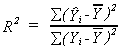and noted that a serious problem with this was that for a given n we can always bring R2 closer to 1 by throwing in more variables. A solution to this problem is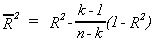This is known as the adjusted R2. The researcher pays a penalty for throwing in extraneous variables.
Some people use the adjusted R2 as a selection criterion for additional independent variables. This is a poor practice since the adjusted R2 is order sensitive. That is, if you put the variables together in a different order you may find the adjusted R2 going up with the addition of Xj only a small amount under one order and a lot under another.
Some Algebraic and Geometric Insights into OLS

1. From the normal equations:

X'Xb-X'y=0
or
X'(y-Xb)=0
We recognize y-Xb as the discrepancy or residual vector. So, for every column of X we have xk'e=0.
i. This means that the regression hyperplane passes through the origin.
ii. Since the first column of X is ordinarily a column of ones it means that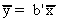.

In ii. the left hand side is the mean of the actual values and the RHS is the mean of the fitted values. They are equal, on average.

2. Projection matrices

By definition e = y-Xb
substitute for b, the least squares estimator
e=y-X(X'X)-1X'y
e=(In-X(X'X)-1X')y
e=My
We can see, from above, that MX=0. Therefore, M and X span subspaces that are orthogonal complements.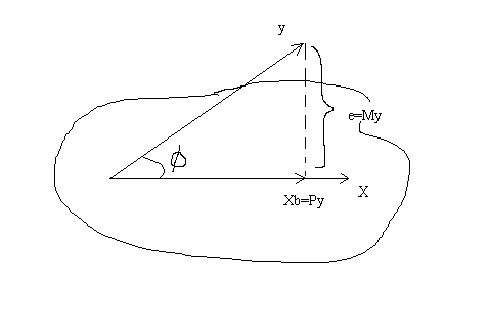We can see that y is composed of the combination of two orthogonal components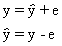By substitution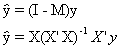so P=X(X'X)-1X' is the matrix which projects y onto the subspace spanned by X. And M=I-P is the matrix which projects y onto the orthogonal complement of X.

The rank of X is k, the rank of In is n. Therefore, (P)=k and the rank of M=n-k.

THEOREM (Davidson and Mackinnon, P. 11-12) Invariance
The fitted values of y and the residuals are invariant to nonsingular, linear transformations of the matrix of regressors.
Proof: Results from the invariance of the subspace spanned by X. The importance is that it doesn't really matter where we put the decimal in our RHS data if our only interest is forecasting. In fact, from a computational and rounding error standpoint, it pays to rescale the data in your model to indices whenever the units of measurement are markedly different.

3. Goodness of Fit

If we plug our best guess for the coefficients into the objective function, then the sum of squared residuals is

(y-Xb)'(y-Xb)= e'e
= (y-X(X'X)-1X'y)'(y-X(X'X)-1X'y)
= y'MMy
= y'My
= My 2 the length of the residual vector.

Expanding the LHS of the first line

e'e=y'y-2b'X'y+b'X'Xb
for the middle term we can write
-2b'X'y = -2y'X(X'X)-1X'y
= -2[y'X(X'X)-1](X'X)[(X'X)-1X'y]
so
e'e = y'y-b'X'Xb
or
y'y=b'X'Xb+e'e

So we can offer the following
b'X'Xb = (y'X(X'X)-1X')(X(X'X)-1X'y)
= (Py)'(Py)
= Py2 , the length of the fitted vector.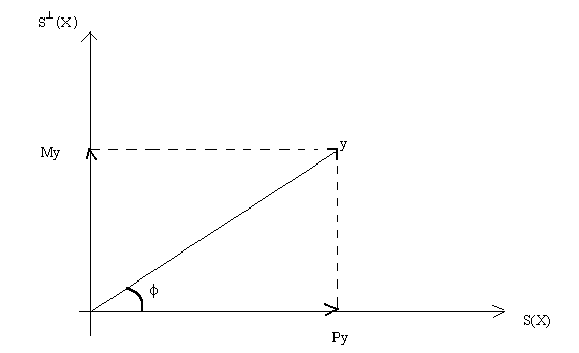So the conclusion is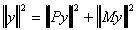This lends itself to an interpretation of goodness of fit. If the discrepancy between y and the fitted vector is quite small then the length of the residual vector will be quite short. Or, the length of the fitted vector will be quite close to the length of y. A natural definition then is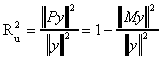where the subscript u denotes "uncentered", the meaning of which will become clear in a moment.
From a bit of trigonometry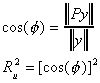But we have a problem. Suppose we add a constant to each element in the vector y. Consider a specific example: The model is y=x1b1+x2b2+u. Our original data is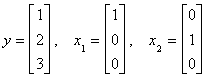. Now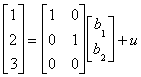. Applying our least squares estimator, b=(X'X)-1X'y.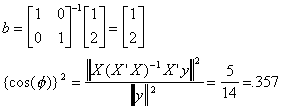But suppose we add 1 to each observation on y so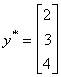, but X is unchanged. Then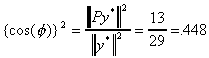.

We have raised the goodness of fit measure by the simple expedient of adding a constant to each observation on y! Note that if we multiply y by a scalar there would be no impact on the goodness of fit measure, since it merely lengthens y but does not displace it in the n-dimensional space (See the following picture).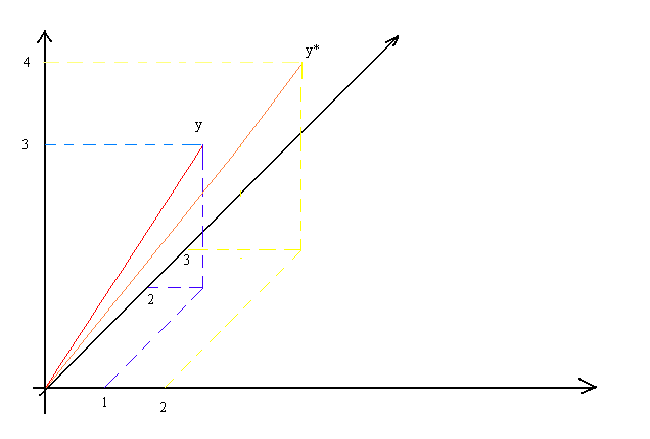Since x1 and x2 are the first two vectors in the orthonormal basis you should be able to sketch them in. Also sketch in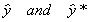. You can now see why the Ru2 has gone up.

In the Bread and Meat exercise set you had to take logs of meat consumption. The problem was that in some cases meat consumption was zero. One suggested fix up was that you add a small number to each observation. You now can see the consequence of doing this.

To get around the problem we measure y as a deviation from its mean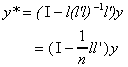and calculate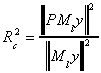And the moral of the story is that you must always include a constant.

4. Partitioned Regression

Suppose that we are entertaining the model y = X + u, which we are able to partition as y = X11 + X22 + u, in which X1 and X2 are nxk1 and nxk2, respectively. The normal equations are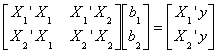with some algebra
b1 = (X1'X1)-1X1'y - (X1'X1)-1X1'X2b2
The first term on the RHS is the projection of y onto the space spanned by the columns in X1. Looking more closely at the 2nd term,(X1'X1)-1X1'X2 is the projection of each of the columns of X2 onto the space spanned by X1. We can represent this in the following diagram: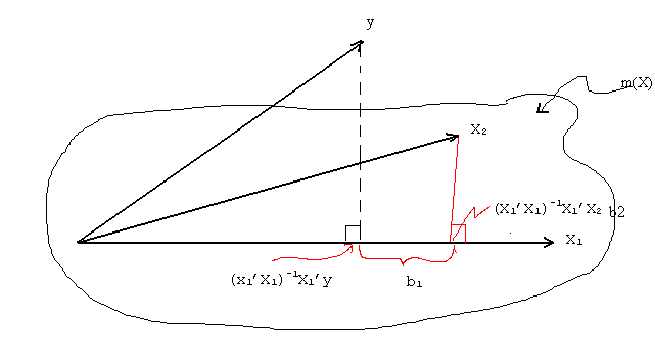In the figure X1 and x2 span a k1+k2 dimensional space denoted by m(X), and which looks like a lake or artist's palette.
With more algebra
b2 = [X2'(I-X1'(X1'X1)-1X1')X2]-1 (X2'(I-(X1'X1)-1X1'))y
To interpret this consider the following
Step 1. Regress y on X1 alone and save the residuals to get

y = X11 + u1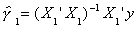and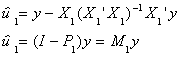Step 2. Regress each of the columns of X2 on the set of regressors X1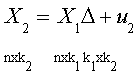Note the dimensions are shown. So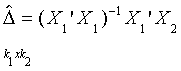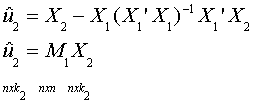Step 3. Now let us run the regression model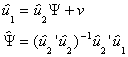by substitution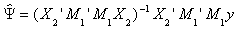by idempotency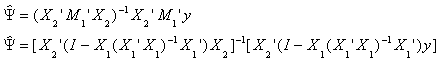which we know to be b2.

Another important result is that the residuals from direct estimation of the original model and the residuals at the final step of the partitioned model will be the same.

Implications:

1. The result presented in the figure is known as the Waugh-Frisch-Lovell Theorem (WFL). The main result is that it permits the "ceteris paribus" interpretation of coefficients.
2. It means detrending and deseasonalizing the the data (with dummies) first is equivalent to including a time trend and seasonal dummies on the RHS.
3. At the time of programming it can economize on computation.